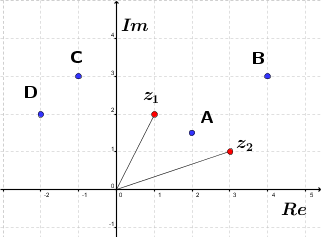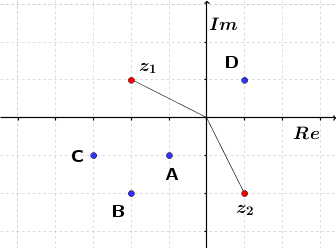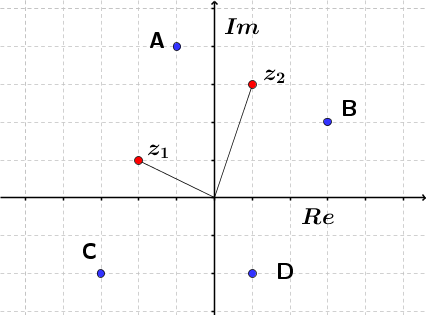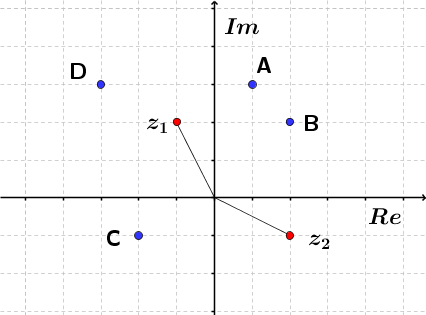Math Calculators, Lessons and Formulas

It is time to solve your math problem

mathportal.org
• Complex Numbers
• Tests in Complex Numbers
• Adding and subtracting complex numbers

# Adding and subtracting complex numbers

ans:
syntax error
C
DEL
ANS
±
(
)
÷
×
7
8
9
4
5
6
+
1
2
3
=
0
.
auto next question
calculator
•  Question 1: 1 pts Add: $(2 + 3i) + (3-4i)$
 $5+7i$ $5-7i$ $5+i$ $5-i$
•  Question 2: 1 pts Add: $(-5-11i) + (4-6i)$
 $1-5i$ $1-17i$ $-1-17i$ $-1-5i$
•  Question 3: 1 pts Subtract: $(5-3i) - (3-2i)$
 $2-i$ $2+i$ $2-5i$ $2+5i$
•  Question 4: 1 pts Subtract: $(-1-i) - (3-5i)$
 $4-4i$ $-4+4i$ $-2+4i$ $2-4i$
•  Question 5: 2 pts Simplify expression $2(1-i) - 3(3-5i)$
 $-11+17i$ $-7-13i$ $-7+13i$ $11-17i$
•  Question 6: 2 pts Simplify expression $-3(5-2i) - 4(2-i)$
 $-23+10i$ $-23-10i$ $17+10i$ $17-10i$
•  Question 7: 2 pts Which point represents the sum of complex numbers $z1$ and $z2$.A B C D
•  Question 8: 2 pts Which point represents the sum of complex numbers $z1$ and $z2$.A B C D
•  Question 9: 2 pts Which point represents the difference of complex numbers $z1$ and $z2$.A B C D
•  Question 10: 2 pts Which point represents the difference of complex numbers $z1$ and $z2$.A B C D